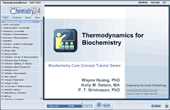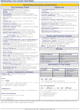How to Learn in 24 Hours?

 Need Help? M-F: 9am-5pm(PST): Toll-Free: (877) RAPID-10 or 1-877-727-4310 24/7 Online Technical Support: The Rapid Support Center vip@rapidlearningcenter.com Secure Online Order:Need Proof? Testimonials by Our Users

 Rapid Learning Courses: MCAT in 24 Hours (2021-22) USMLE in 24 Hours (Boards) Chemistry in 24 Hours Biology in 24 Hours Physics in 24 Hours Mathematics in 24 Hours Psychology in 24 Hours SAT in 24 Hours ACT in 24 Hours AP in 24 Hours CLEP in 24 Hours DAT in 24 Hours (Dental) OAT in 24 Hours (Optometry) PCAT in 24 Hours (Pharmacy) Nursing Entrance Exams Certification in 24 Hours eBook - Survival Kits Audiobooks (MP3)

 Tell-A-Friend: Have friends taking science and math courses too? Tell them about our rapid learning system.Home »  Chemistry »  Biochemistry

Thermodynamics for Biochemistry

 Topic Review on "Title": Thermodynamics It is the study of heat changes. Increased temperature increases molecular motion. Energy is the ability to do work. Joules (J) and calories (cal) are the most common energy units. Energy can be transferred through work applying a force over a distance. Energy changes of physical and chemical processes First law of thermodynamics states that energy can not be created or destroyed in physical or chemical changes. Heat is the flow of energy from higher temperature particles to lower temperature particles. Enthalpy, entropy and free energy Enthalpy (H) takes into account the internal energy of the sample along with pressure and volume. Under constant pressure, heat and enthalpy are the same. Enthalpy of reaction is the net energy change during a chemical reaction. In endothermic reaction, the system takes in energy from the surroundings. ΔD is positive. In exothermic reaction, the system gives off energy to the surroundings. Here, ΔH is negative. Heat capacity is the amount of energy that can be absorbed by a particle, before the temperature increases. When changing between solids, liquids and gases, the temperature does not change. Entropy is the randomness. Spontaneity occurs without outside intervention. Second law of thermodynamics states that every spontaneous process has an increase in entropy of the universe. Determining spontaneity of a process Spontaneity occurs without outside intervention. Temperature can determine whether a process is spontaneous. Free energy (G) relates temperature, enthalpy and entropy. Free energy is used to determine if the reaction is spontaneous at a specific temperature. Relating free energy and equilibrium  A chemical reaction that can proceed in both directions is called a reversible reaction. A reaction is said to be at equilibrium when the rate of the forward reaction equals the rate of the reverse reaction. It takes time to establish equilibrium. The reactions continue to proceed in both directions, but at the same rate is said to be in dynamic equilibrium. At thermal equilibrium, two objects at different temperatures, placed together will come to the same temperature.

Rapid Study Kit for "Title":
 Flash Movie Flash Game Flash Card Core Concept Tutorial Problem Solving Drill Review Cheat Sheet"Title" Tutorial Summary : It is the study of heat changes. Increased temperature increases molecular motion. Energy is the ability to do work. Joules (J) and calories (cal) are the most common energy units. Under constant pressure, heat and enthalpy are the same. When changing between solids, liquids and gases, the temperature does not change. Entropy is the randomness. Spontaneity occurs without outside intervention. Free energy (G) relates temperature, enthalpy and entropy. When the rate of forward reaction equals rate of reverse reaction, a state of equilibrium is reached.

 Tutorial Features: Concept map to explain thermodynamics. Concept map to explain equilibrium. Brief definitions with suitable examples are given. Simplified derivatives for dissociation constants.

 "Title" Topic List: Thermodynamics Introduction Definition Energy changes of physical and chemical processes 1st law of thermodynamics System and surroundings Energy and its units Energy and work Enthalpy, entropy and free energy Heat and enthalpy Enthalpy of reaction Endothermic and exothermic reactions Heating curves Heat capacity Change in temperature and state 2nd law of thermodynamics Entropy Determining spontaneity of a process Temperature and spontaneity Summary of spontaneous conditions Relating free energy and equilibrium Definition of free energy Free energy and spontaneity Free energy example Definition of equilibrium Dynamic equilibrium Thermal equilibrium Free energy and equilibrium constants Free energy at non standard conditions Definition of equilibrium constant Expression of equilibrium constant Heterogeneous equilibrium

See all 24 lessons in college chemistry, including concept tutorials, problem drills and cheat sheets:
Teach Yourself Biochemistry Visually in 24 Hours